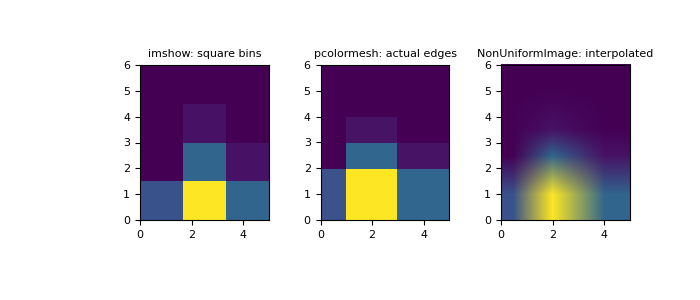# numpy.histogram2d¶

`numpy.``histogram2d`(x, y, bins=10, range=None, normed=None, weights=None, density=None)[source]

Compute the bi-dimensional histogram of two data samples.

Parameters
xarray_like, shape (N,)

An array containing the x coordinates of the points to be histogrammed.

yarray_like, shape (N,)

An array containing the y coordinates of the points to be histogrammed.

binsint or array_like or [int, int] or [array, array], optional

The bin specification:

• If int, the number of bins for the two dimensions (nx=ny=bins).

• If array_like, the bin edges for the two dimensions (x_edges=y_edges=bins).

• If [int, int], the number of bins in each dimension (nx, ny = bins).

• If [array, array], the bin edges in each dimension (x_edges, y_edges = bins).

• A combination [int, array] or [array, int], where int is the number of bins and array is the bin edges.

rangearray_like, shape(2,2), optional

The leftmost and rightmost edges of the bins along each dimension (if not specified explicitly in the bins parameters): `[[xmin, xmax], [ymin, ymax]]`. All values outside of this range will be considered outliers and not tallied in the histogram.

densitybool, optional

If False, the default, returns the number of samples in each bin. If True, returns the probability density function at the bin, `bin_count / sample_count / bin_area`.

normedbool, optional

An alias for the density argument that behaves identically. To avoid confusion with the broken normed argument to `histogram`, density should be preferred.

weightsarray_like, shape(N,), optional

An array of values `w_i` weighing each sample `(x_i, y_i)`. Weights are normalized to 1 if normed is True. If normed is False, the values of the returned histogram are equal to the sum of the weights belonging to the samples falling into each bin.

Returns
Hndarray, shape(nx, ny)

The bi-dimensional histogram of samples x and y. Values in x are histogrammed along the first dimension and values in y are histogrammed along the second dimension.

xedgesndarray, shape(nx+1,)

The bin edges along the first dimension.

yedgesndarray, shape(ny+1,)

The bin edges along the second dimension.

`histogram`

1D histogram

`histogramdd`

Multidimensional histogram

Notes

When normed is True, then the returned histogram is the sample density, defined such that the sum over bins of the product `bin_value * bin_area` is 1.

Please note that the histogram does not follow the Cartesian convention where x values are on the abscissa and y values on the ordinate axis. Rather, x is histogrammed along the first dimension of the array (vertical), and y along the second dimension of the array (horizontal). This ensures compatibility with `histogramdd`.

Examples

```>>> from matplotlib.image import NonUniformImage
>>> import matplotlib.pyplot as plt
```

Construct a 2-D histogram with variable bin width. First define the bin edges:

```>>> xedges = [0, 1, 3, 5]
>>> yedges = [0, 2, 3, 4, 6]
```

Next we create a histogram H with random bin content:

```>>> x = np.random.normal(2, 1, 100)
>>> y = np.random.normal(1, 1, 100)
>>> H, xedges, yedges = np.histogram2d(x, y, bins=(xedges, yedges))
>>> H = H.T  # Let each row list bins with common y range.
```

`imshow` can only display square bins:

```>>> fig = plt.figure(figsize=(7, 3))
>>> ax = fig.add_subplot(131, title='imshow: square bins')
>>> plt.imshow(H, interpolation='nearest', origin='lower',
...         extent=[xedges, xedges[-1], yedges, yedges[-1]])
<matplotlib.image.AxesImage object at 0x...>
```

`pcolormesh` can display actual edges:

```>>> ax = fig.add_subplot(132, title='pcolormesh: actual edges',
...         aspect='equal')
>>> X, Y = np.meshgrid(xedges, yedges)
>>> ax.pcolormesh(X, Y, H)
```

`NonUniformImage` can be used to display actual bin edges with interpolation:

```>>> ax = fig.add_subplot(133, title='NonUniformImage: interpolated',
...         aspect='equal', xlim=xedges[[0, -1]], ylim=yedges[[0, -1]])
>>> im = NonUniformImage(ax, interpolation='bilinear')
>>> xcenters = (xedges[:-1] + xedges[1:]) / 2
>>> ycenters = (yedges[:-1] + yedges[1:]) / 2
>>> im.set_data(xcenters, ycenters, H)
>>> ax.images.append(im)
>>> plt.show()
```